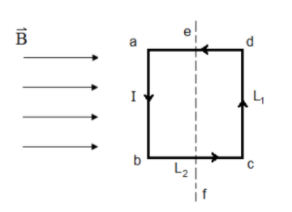# Problem: A rectangular loop with L1 = 0.15 m and L2 = 0.35 m is sitting in a magnetic field B = 0.35 T as shown in the figure. There is a counterclockwise current I = 0.55 A in the loop. Part (a) Express the magnitude of the magnetic force on ab or cd, Fl, in terms of the length Ll, current I and magnetic field B.Fl =Part (b) Calculate the numerical value of the magnitude of the force, Fl, on ab or cd, in N.Part (c) Express the magnitude of the magnetic force on bc or ad, F2, in terms of the length L2, current I and magnetic field B.

###### FREE Expert Solution

Part (a)

F1 = iBL1sin(90°) = iBL1

85% (64 ratings)###### Problem Details

A rectangular loop with L1 = 0.15 m and L2 = 0.35 m is sitting in a magnetic field B = 0.35 T as shown in the figure. There is a counterclockwise current I = 0.55 A in the loop.Part (a) Express the magnitude of the magnetic force on ab or cd, Fl, in terms of the length Ll, current I and magnetic field B.

Fl =

Part (b) Calculate the numerical value of the magnitude of the force, Fl, on ab or cd, in N.

Part (c) Express the magnitude of the magnetic force on bc or ad, F2, in terms of the length L2, current I and magnetic field B.